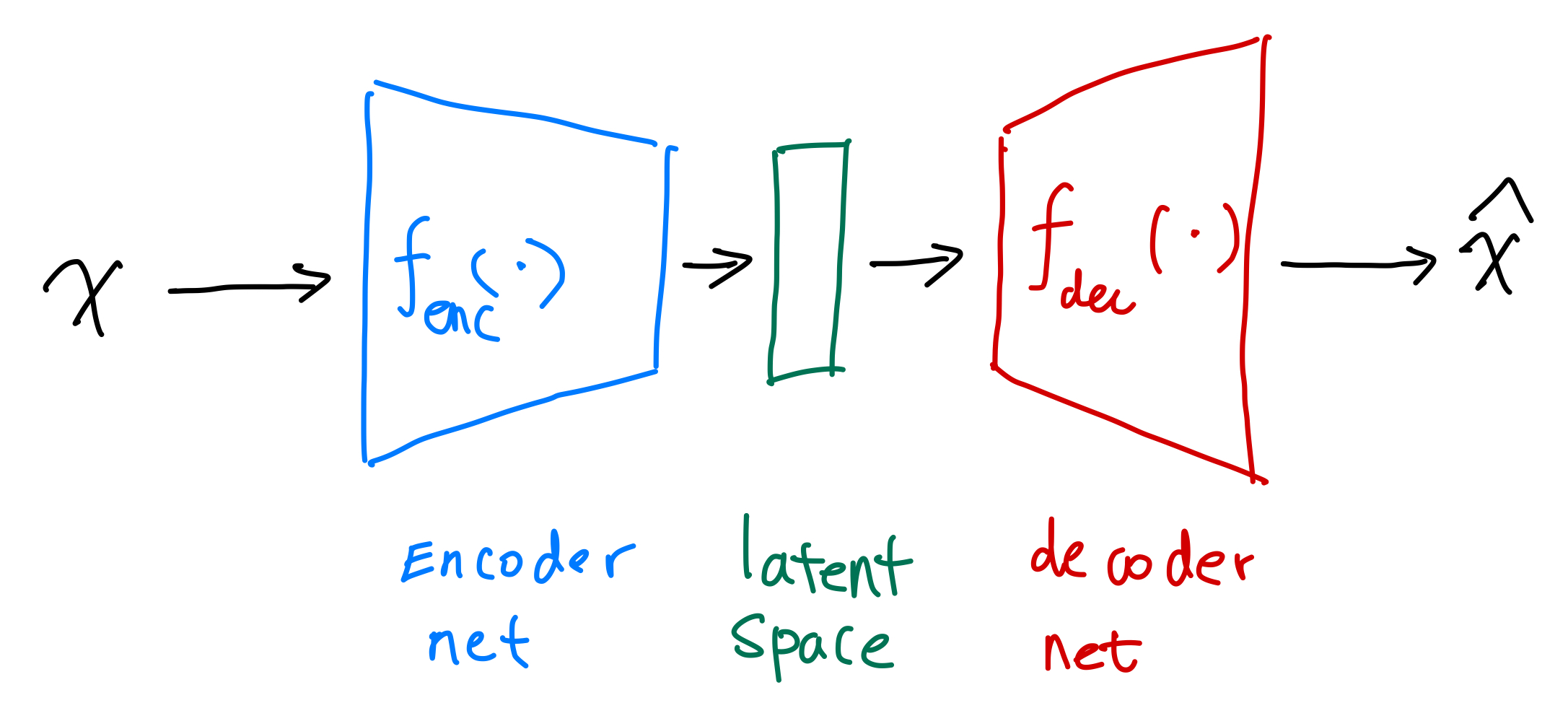# Autoencoders¶

Autoencoders (AE) are machines that encode inputs into a compact latent space.Notation: dot ($$\cdot$$)

We use a single vertically centered dot, i.e., $$\cdot$$, to indicate that the function or machine can take in arguments.

A simple autoencoder can be achieved using two neural nets, e.g.,

\begin{align} {\color{green}h} &= {\color{blue}g}{\color{blue}(}{\color{blue}b} + {\color{blue}w} x{\color{blue})} \\ \hat x &= {\color{red}\sigma}{\color{red}(c} + {\color{red}v} {\color{green}h}{\color{red})}, \end{align}

where in this simple example,

• $${\color{blue}g(b + w \cdot )}$$ is the encoder, and
• $${\color{red}\sigma(c + v \cdot )}$$ is the decoder.

For binary labels, we can use a simple cross entropy as the loss.

## Code¶

See Lippe1.

1. Lippe P. Tutorial 9: Deep Autoencoders — UvA DL Notebooks v1.1 documentation. In: UvA Deep Learning Tutorials [Internet]. [cited 20 Sep 2021]. Available: https://uvadlc-notebooks.readthedocs.io/en/latest/tutorial_notebooks/tutorial9/AE_CIFAR10.html

Contributors: LM